# Math Equations

Step by Step

## Overview

The WCMS WYSIWYG Editor now supports the <MATH> tag in HTMLexternal icon. This means that you can use Microsoft Word (or any editor that generates the math code such as MathML) to create mathematical equations and then copy them into the WCMS.  Note that MathML (from Microsoft Word) supports interactivity. Click the equation below to enlarge the view.  Right-click to access MathML equation settings, such as the zoom factor.

$x+an=∑k=0nnkxkan-k$

Below are the general instructions for working with equations using a Word .doc file as the source document.

## Creating an Equation

1. In Microsoft Word, open the Insert menu from the menu bar.
2. To insert a blank equation container, click the “Equation” option. To select a built-in equation format, open the equation gallery by clicking the down arrow to the right of the “Equation” option.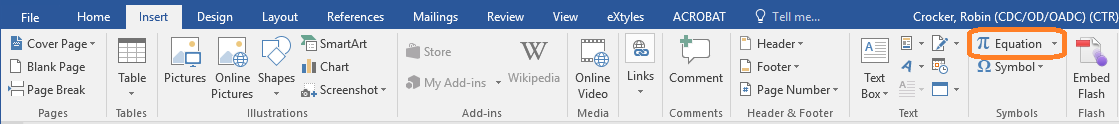Once your equation is in place, you can edit it directly in the equation container: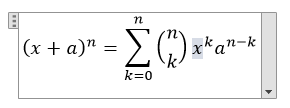With the cursor in the equation container, you can also open the Design menu to access equation tools and options: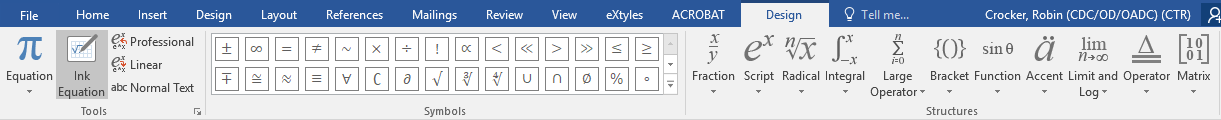## Copying the Equation Code

1. From the Design menu, open the equation Tools settings: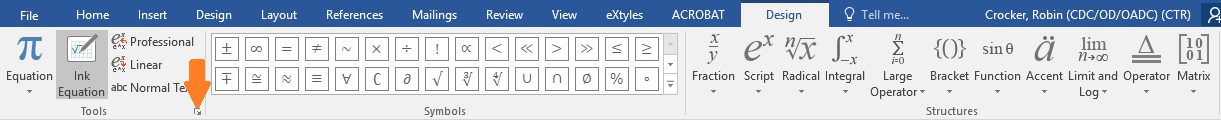1. Make certain the following option is turned on:  “Copy MathML to the clipboard as plain text.”
2. Select the equation and copy it. (Tip: To select the whole equation, click the small tab on the left side of the equation container.)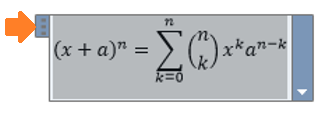## Pasting into the WCMS

Content owners who have access to the WCMS can paste the MathML code directly into a WCMS text block, text box, etc. Otherwise, the content owner must provide the source code to a WCMS developer.

To insert the code, position the cursor in the text and select the “Insert Equation” tool in the WCMS WYSIWYG Editor. (Tip: When inserted inline, a tall equation may cause inconsistencies in paragraph line spacing. In this case, consider placing the equation on its own line.)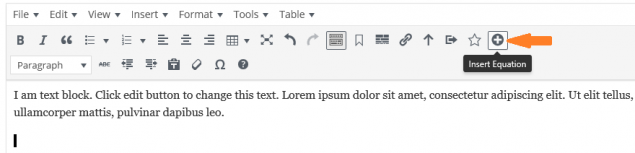Then paste the code when prompted: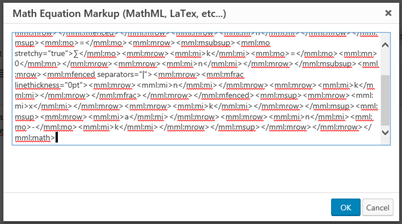Page last reviewed: May 31, 2019